# Linear algebra for game developers ~ part 4

July 31st, 2010

Last time we talked about transformation matrices, and how they let us change from one coordinate system to another. A lot of you expressed interest in quaternions, so today I will go over 3D rotations. But first let's review 2D rotations.

## 2D Rotation

Since there is only one possible axis of rotation (the axis going into the screen), the only information we need is the angle. I mentioned last time that using trigonometry we can create a 2D rotation function like this:

```vec2 rotate(vec2 point, float angle){
vec2 rotated_point;
rotated_point.x = point.x * cos(angle) - point.y * sin(angle);
rotated_point.y = point.x * sin(angle) + point.y * cos(angle);
return rotated_point;
}
```

This is more elegantly expressed in matrix form. To find the matrix version, we can apply the function to the axes (1,0) and (0,1) for angle θ, and then plug the new axes into the columns of our matrix. Let's start with the x axis: (1,0). If we plug it into our function we get:

(1*cos(θ) - 0*sin(θ), 1*sin(θ) + 0*cos(θ)) = (cos(θ), sin(θ))

Next, we plug in the y axis: (0,1). This gives us:

(0*cos(θ) - 1*sin(θ), 0*sin(θ) + 1*cos(θ)) = (-sin(θ), cos(θ))

Plugging these new axes into the columns of a matrix gives us our 2D rotation matrix:

```[cos(θ) -sin(θ)
sin(θ)  cos(θ)]```

Let's apply this matrix to Suzanne, the Blender monkey, with θ = 45 degrees clockwise.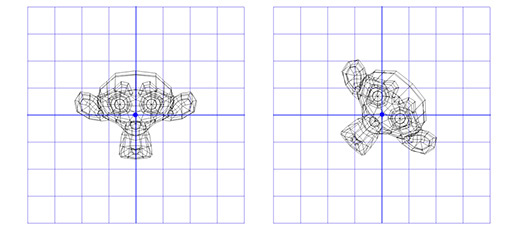It works! But what if we want to rotate around a point that is not (0,0)? For example, maybe we want the monkey to rotate around a point on its ear, like this: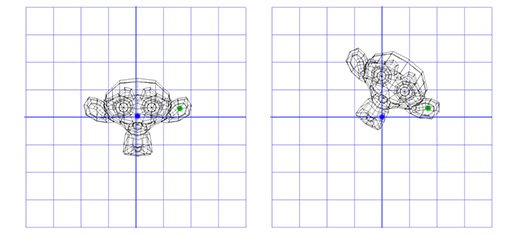To do this, we can start by making a translation matrix T that moves from the origin to the ear pivot point, and a rotation matrix R that rotates around the origin. Now, to rotate around the ear pivot point, we can first move the ear pivot point to the origin with the inverse of T, written T-1. Then we just rotate around the origin using R, and then apply T to move the pivot point back to its original position. Here is an illustration of each step: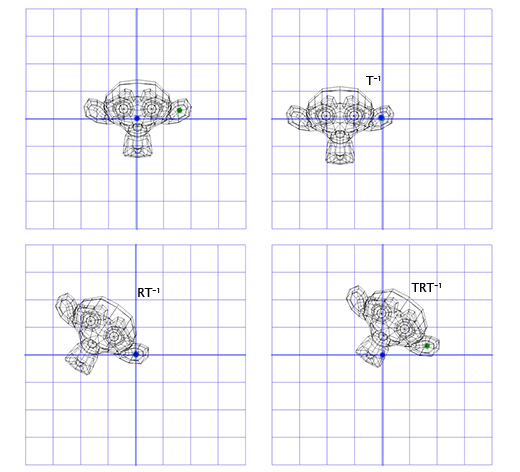This is an important pattern that we will use again later -- putting the rotation within two opposite transformations lets us do the rotation in a different 'space', which is very useful. Now, let's look at 3D rotation.

## 3D Rotation

Rotation about the Z axis works just like it did in 2D. We just have to adapt our old matrix by adding an extra column and row:

```[cos(θ) -sin(θ) 0
sin(θ)  cos(θ) 0
0       0      1]```

Let's apply this matrix to the 3D version of Suzanne, with θ = 45 degrees clockwise.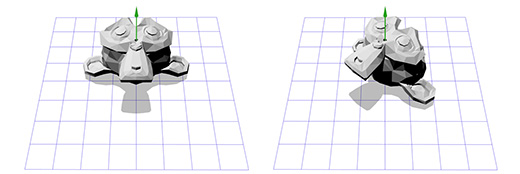Pretty much the same so far! It's pretty limiting to just rotate around the z-axis though -- maybe we want to rotate around any axis we want.

### Axis-angle rotations

The axis-angle rotation format represents rotations like this: (axis, angle). Simple enough! This format is very useful, and is the starting point of many of the rotations I work with. But how do we actually apply an axis-angle rotation? Let's say we have a weird axis like this one: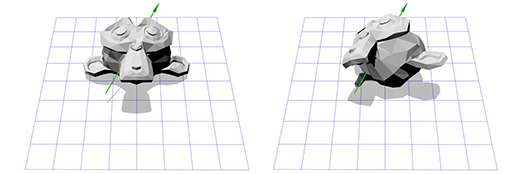That seems tricky -- but we already have the tools to do it. We know how to rotate around the z-axis, and we know how to rotate in different spaces. So, we just have to create a new space where this axis is the z-axis! But if this axis is the z-axis, what are the x and y axes? That's what we have to construct now.

To create the x and y axes, we just have to pick two vectors that are perpendicular to the new z-axis, and perpendicular to each other. Fortunately for us, we talked about the 'cross product' in part two, which inputs two vectors and outputs a perpendicular one. We only have one vector so far, the rotation axis -- let's call it A. Now we can just pick a vector B at random, as long as it's not in the same direction as A. Let's pick (0,0,1) for convenience.

Now that we have the rotation axis A and our random vector B, we can get the normalized cross product, C, which is perpendicular to both other vectors. Now we can make B perpendicular by setting it to the cross product of A and C, and we have our axes!

This sounds complicated when put in words, but it's actually really simple in code or in images. Here's how it would look in code:

```B = (0,0,1);
C = cross(A,B);
B = cross(C,A);```

Here is an illustration of each step :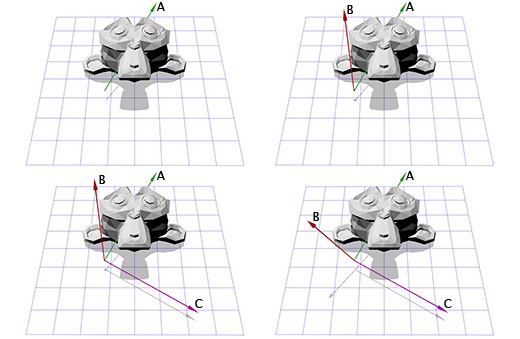Now that we have our new axes, we can create a matrix M by plugging in each axis into a column. We have to make sure that vector A is in the third column in order to make it the new z-axis:

```[B0 C0 A0
B1 C1 A1
B2 C2 A2]```

Now it's like what we did for the ear pivot in 2D. We can apply the inverse of M to move into our new coordinate space, then apply rotation R to rotate around the z axis, and then apply M to move back into our usual space.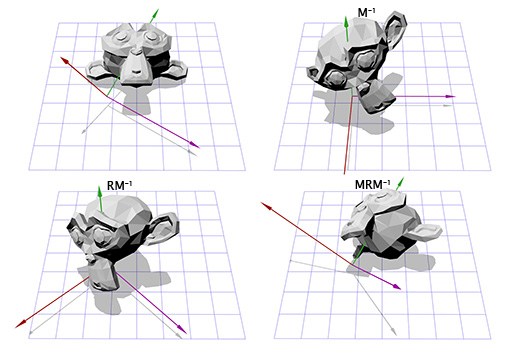If that made sense to you, then congratulations, you can rotate around any axis! After all that work, we can just create a matrix T = M-1RM and use it as many times as we like without any further effort. There are more efficient ways to convert axis-angle rotations to matrix rotations, but this way covers a lot of what we've been talking about.

Axis-angle rotations are perhaps the most intuitive rotation format. They are also the easiest to invert (switch the sign of the angle), and the easiest to interpolate (just interpolate the angle). However, they have a serious limitation in that they cannot accumulate. That is, you can't combine two axis-angle rotations into a third. Axis-angle rotations are a good starting place, but they have to be turned into something else to be used for anything complicated.

### Euler angles

Euler angles (pronounced 'oyler') are another common rotation format, consisting of three nested rotations around the x, y and z axes. They are probably most familiar to you from the standard first-person or third-person game cameras.

Let's say you're in an FPS game and you rotate 30 degrees left, and then look 40 degrees up. Finally, someone shoots you, and the impact effect rolls the camera on its axis 45 degrees. Here's an illustration of this ZYX euler angle (30, 40, 45)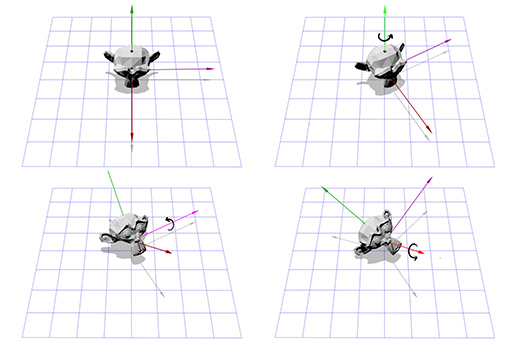Euler angles are convenient because they're intuitive and easy to control. However, they do have two drawbacks which limit their use:

First, it's possible to reach a situation called 'gimbal lock'. Imagine you are in the first-person shooter we just described, where you can look left, right, up and down, or roll the camera on its axis. Now imagine you are looking straight up. In this situation, looking left or right is the same thing as rolling the camera -- all we can do is roll the camera or look down! As you can imagine, this limitation makes Euler angles impractical for flight simulators.

Second, interpolating between two Euler angle rotations does not give the shortest path between them. For example, here are two interpolations between the same rotations. The first is using Euler angle interpolation, and the second is using spherical linear interpolation (SLERP) to find the shortest path.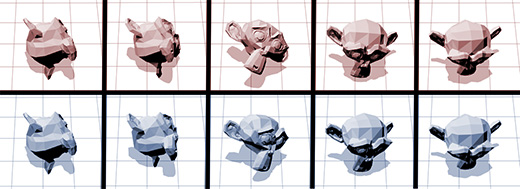So what is good for interpolating rotations? Would matrices work better?

### Matrix rotations

As we've discussed, rotation matrices just store the three axes. This means that interpolating between two matrices just linearly interpolates each axis. This does result in an efficient path, but also introduces other problems. For example, here are two rotations and an interpolated half rotation: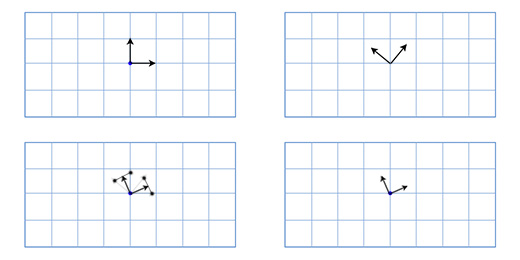As you can see, the interpolated rotation is significantly smaller than either of the source rotations, and the two axes are no longer perfectly perpendicular. This makes sense if you think about it -- if you average the position of any two points on a sphere, then you will end up closer to the center. This problem causes a trademark 'candy wrapper' effect when used for skeletal animation. Here's an Overgrowth rabbit demonstrating this effect: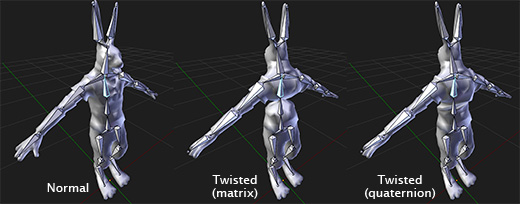Matrix rotations are very useful in that they can accumulate without any problems like gimbal lock, and can most efficiently be applied to points. This is why they are used natively on graphics cards -- for any kind of 3D graphics, the matrix format is always the end goal.

However, as we've seen, they do not interpolate well, and they are not very intuitive to create in many cases.

Well, there's only one major rotation format left. Last but not least, we have:

### Quaternions

So what are quaternions? The quick and dirty answer is they are an alternate form of axis-angle rotations that lives in 'rotation space'. Like matrices, they can accumulate -- that is, you can chain them together indefinitely without any problems like gimbal lock. However, unlike matrices, they can smoothly interpolate from one orientation to another.

Are quaternions just better than all the other rotation formats? So far, they seem to combine all of their strengths. Unfortunately, they have two major weaknesses that ensure that they are only useful as intermediate rotations. First, they have no easy mapping to 3D space, so you will always create rotations in a more user-friendly format and convert it over. Second, they cannot efficiently rotate points, so you have to convert them to matrices in order to rotate significant numbers of points.

This means you will likely never start or end a series of rotations with a quaternion, but they can do intermediate processing more efficiently than any other format.

The internal workings of quaternions are not really intuitive or interesting to me, and probably won't be for you either if you're not a mathematician, so I advise finding libraries or functions online that wrap quaternions in a way that makes them easy to use. The Bullet or Blender math libraries could be a good place to start, and there are many others on the Internet.